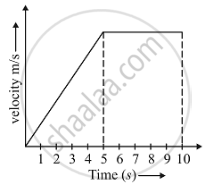Share

# Draw a Velocity-time Graph to Show the Following Motion : a Car Accelerates Uniformly from Rest for 5 S ; Then It Travels at a Steady’ Velocity for 5 S. - CBSE Class 9 - Science

ConceptGraphical Representation of Motion - Velocity-time Graphs

#### Question

Draw a velocity-time graph to show the following motion :
A car accelerates uniformly from rest for 5 s ; then it travels at a steady’ velocity for 5 s.

#### Solution

we have to draw a velocity verses time curve for a moving body .Is there an error in this question or solution?

#### APPEARS IN

Solution Draw a Velocity-time Graph to Show the Following Motion : a Car Accelerates Uniformly from Rest for 5 S ; Then It Travels at a Steady’ Velocity for 5 S. Concept: Graphical Representation of Motion - Velocity-time Graphs.
S